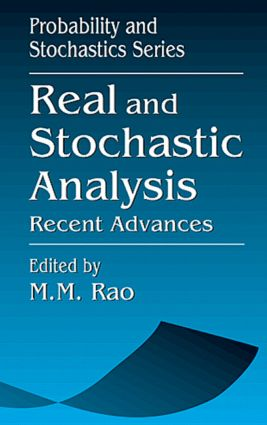Real and Stochastic AnalysisRecent Advances

1st Edition

CRC Press

416 pages

Purchasing Options:\$ = USD
Hardback: 9780849380785
pub: 1997-03-06
SAVE ~\$37.00
Currently out of stock
\$185.00
\$148.00
x

FREE Standard Shipping!

Description

Real and Stochastic Analysis: Recent Advances presents a carefully edited collection of research articles written by research mathematicians and highlighting advances in RSA. A balanced blend of both theory and applications, this book covers six aspects of stochastic analysis in depth and detail.

The first chapters cover the state of the art in tracers analysis, stochastic modeling as it applies to AIDS epidemiology, and the current state of higher order SDEs. Subsequent chapters present a simple approach to Gaussian dichotomy, an overview of harmonizable processes, and stochastic Fubini and Green theorems. Common to all the chapters, the employment of functional analytic methods creates a unified approach. Each chapter includes detailed proofs.

Throughout the book, a substantial amount of new material is presented, much of it for the first time. This forward-looking work presents current accounts of important areas of research, evaluates recent advances, and identifies research frontiers and new challenges.

Introduction and Overview, M.M. Rao

Transport Properties of Gaussian Velocity Fields, R.A. Carmona

Introduction

Gaussian Velocity Fields

Infinite-Dimensional Ornstein-Uhlenbeck Processes

Transport Problems

Numerical Simulations

The Case of White Velocity Fields

Open Mathematical Problems

Stochastic Flows and SPDEs

Bibliography

Planar Stochastic Integration Relative to Quasimartingales, M.L. Green

Introduction

The Bochner Bounding Measure for V-Quasimartingales

Line Integrals

The Mixed Integral

The Double Integral

The JX-Measure

A Stochastic Green Theorem

Bibliography

Probability Metrics and Limit Theorems in AIDS Epidemiology, S.T. Rachev, V. Haynatzka, and G. Haynatzki

Introduction

The Monge-Kantorovitch Problem and Probability Metrics

Ideal Metrics

Spread of AIDS Among Drug Users and Probability Metrics

The Spread of AIDS Among Interactive Transmission Groups

Bibliography

Higher Order Stochastic Differential Equations, M.M. Rao

Introduction

Stochastic Integrals and Boundedness Principles

Linear Stochastic Differential Equations

Higher Order Nonlinear Equations

Infinitesimal Characteristics of Solutions

Refined Analysis with Brownian Noise

Operator Representation of the V-Process

Sample Path Behavior for Large Time

Aspects of Stochastic Flows

Multiparameter Analogs and SPDEs

Bibliography

Some Aspects of Harmonizable Processes and Fields, R.J. Swift

Introduction

Preliminaries

Part I: Second Order Nonstationary Processes Including the Harmonizable Class

Part II: Some Classes of Harmonizable Random Fields

Bibliography

On Singularity and Equivalence of Gaussian Measures, N. Vakhania and V. Tarieladze

Definitions and Auxiliary Results

Kakutani's Theorem

Gaussian Measures on R

General Gaussian Measures

The Case of Topological Vector Spaces

Bibliography

Index